# On the 55th day of the journey, python opencv perspective transformation front knowledge contour coordinate points

2022-01-30 14:13:42

This article has participated in  「 Digging force Star Program 」 , Win a creative gift bag , Challenge creation incentive fund .

Python OpenCV 365 Day study plan , Go into the field of image with the eraser . This blog is the third in this series 55 piece .

Before formal study , First the Last one Review the content of the blog , Through a series of contour operations , Get the contour of the target image .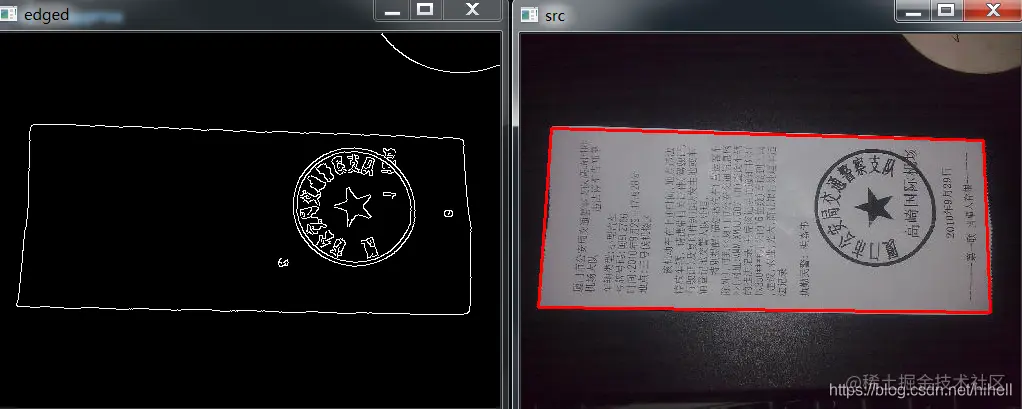The goal of this blog will gradually realize the perspective transformation .

## Get the four vertex coordinates of the contour

Last blog has got the outline coordinates , But the position of each coordinate is uncertain , It needs to be made clear by calculation . The contour coordinates are changed from 3D to 2D

``````cv.drawContours(src, [screen_cnt], -1, (0, 0, 255), 2)

print(screen_cnt)
print(screen_cnt.shape)
print(screen_cnt.reshape(4, 2))
Copy code ``````

The code works as follows , Different pictures get different values .

``````[[[ 30  94]]
[[ 17 273]]
[[469 278]]
[[462 106]]]
(4, 1, 2)
[[ 30  94]
[ 17 273]
[469 278]
[462 106]]
Copy code ``````

After coordinate transformation , The position corresponding to the picture is shown in the figure below .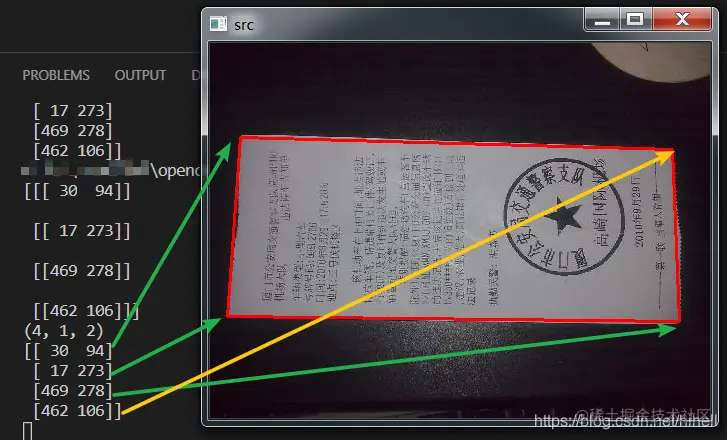In general , We describe a rectangular area , stay OpenCV in , It's using Top left , The upper right , The lower right , The lower left Clockwise order , So next we need to transform these four coordinates .

Declare a function to perform the corresponding calculation .

``````def change_points(input_points):
pass

#  Function call
change_points(screen_cnt.reshape(4, 2))
Copy code ``````

Calculation 4 The sum of the abscissa and ordinate of each point

``````def change_points(input_points):
s = input_points.sum(axis=1)
print(s)
Copy code ``````

The core is numpy Medium `sum` function , Be careful `axis` Shaft parameters ,`0` Vertical means vertical ,`1` It means horizontal , The transverse direction here is the sum of the abscissa and ordinate of the point .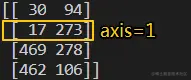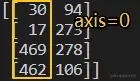For a rectangular area , The upper left point and the lower right point are the point with the smallest sum of abscissa and ordinate and the point with the largest sum of abscissa and ordinate respectively , Based on this , Continue to improve the code .

The following code uses `np.argmin()` and `np.argmax()` function , You can learn quickly through search engines .

``````def change_points(input_points):
s = input_points.sum(axis=1)
p1 = input_points[np.argmin(s)]
p3 = input_points[np.argmax(s)]
print(p1,p3)
Copy code ``````

The data obtained are as follows ：

``````[[ 30  94]
[ 17 273]
[469 278]
[462 106]]
[30 94] [469 278]
Copy code ``````

The lower left point and the upper right point are calculated as , Use `np.diff()` function , Calculate the difference between abscissa and ordinate to find the point in the upper right corner , The difference between abscissa and ordinate is the largest. Find the point in the lower left corner . Corresponding to the figure below can help understand , Lower left `300-100 = 200`, Top right `100-400 = -300`.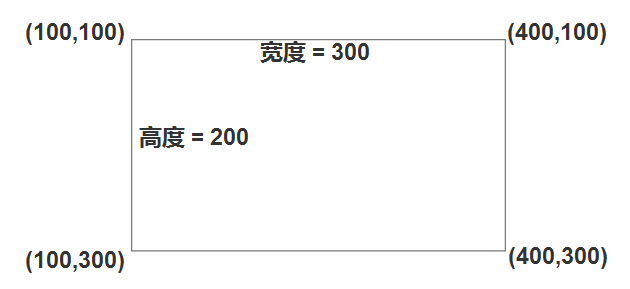``````def change_points(input_points):
s = input_points.sum(axis=1)
p1 = input_points[np.argmin(s)]
p3 = input_points[np.argmax(s)]
print(p1,p3)

diff = np.diff(input_points,axis=1)
p2 = input_points[np.argmin(diff)]
p4 = input_points[np.argmax(diff)]
print(p2,p4)
Copy code ``````

here ,4 All coordinates have been obtained , Finally, declare an empty matrix , The assignment is followed by a return .

``````def change_points(input_points):
s = input_points.sum(axis=1)
p1 = input_points[np.argmin(s)]
p3 = input_points[np.argmax(s)]

diff = np.diff(input_points, axis=1)
p2 = input_points[np.argmin(diff)]
p4 = input_points[np.argmax(diff)]

#  Declare that all elements are  0  Matrix
rect = np.zeros((4, 2), dtype="float32")
rect = p1
rect = p2
rect = p3
rect = p4
print(rect)
return rect
change_points(screen_cnt.reshape(4, 2))
Copy code ``````

The corresponding position of coordinate value and picture is as follows ：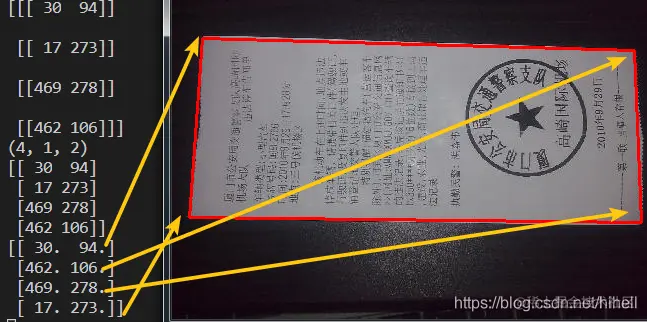## Eraser bars

I hope today's 1 Hours （ It doesn't seem to be enough ） You get something , I'll see you on our next blog ~